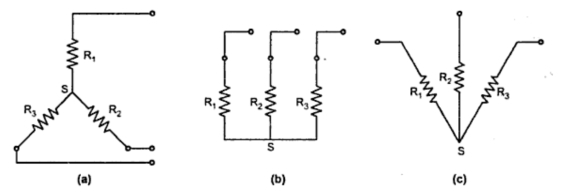### Star and Delta Connection of Resistances

In the complicated networks involving large number of resistances, Kirchhoff's laws give us complex set of simultaneous equations. It is time consuming to solve such set of simultaneous equations involving large number of unknowns. In such a case application of Star-Delta or Delta-Star transformation, considerably reduces the complexity of the network and brings the network into a very simple form. This reduces the number of unknowns and hence network can be analysed very quickly for the required result. These transformations allow us to replace three star connected resistances, without affecting currents in other branches and vice-versa.

Let us see what is star connection ?
If the three resistances are connected in such a manner of that one end of each is connected together to form a junction point called star point, the resistances are said to be connected in Star.
The Fig. 1(a) and (b) show star connected resistances. The star point is indicated as S. Both the connections Fig .1(a) and (b) are exactly identical. The Fig. 1(b) can be redrawn as Fig. 1(a) or vice-versa, in the circuit from simplification point of view.Fig. 1

Let us see what is delta connection ?
If the three resistances are connected in such a manner that one end of the first is connected to first end of second, the second end of second to first end of third and so on to complete a loop then the resistances are said to be connected in Delta.
Key point : Delta connection always forms a loop, closed path.
The Fig. 2(a) and (b) show delta connection of three resistances. The Fig. 2(a) and (b) are exactly identical.Fig 2